# Concise Selina Solutions for Class 9 Maths Chapter 21- Solids

Selina Solutions are useful resources for students as it helps them in scoring great marks in the examination. The Selina Solutions given in this page contains detailed step-by-step explanations to all the problems that come under Chapter 21, Solids, of the Class 9 Selina Textbook. This chapter covers the topics including volume, surface area and total surface area of Solids.

These solutions are prepared by subject matter experts at BYJU’S, explaining the method of solving problems, right from the beginning till the end. By understanding the concepts used in Selina Solutions for Class 9 Maths, students will be able to clear all their doubts related to “Solids”.

## Download PDF of Selina Solutions for Class 9 Maths Chapter 21:-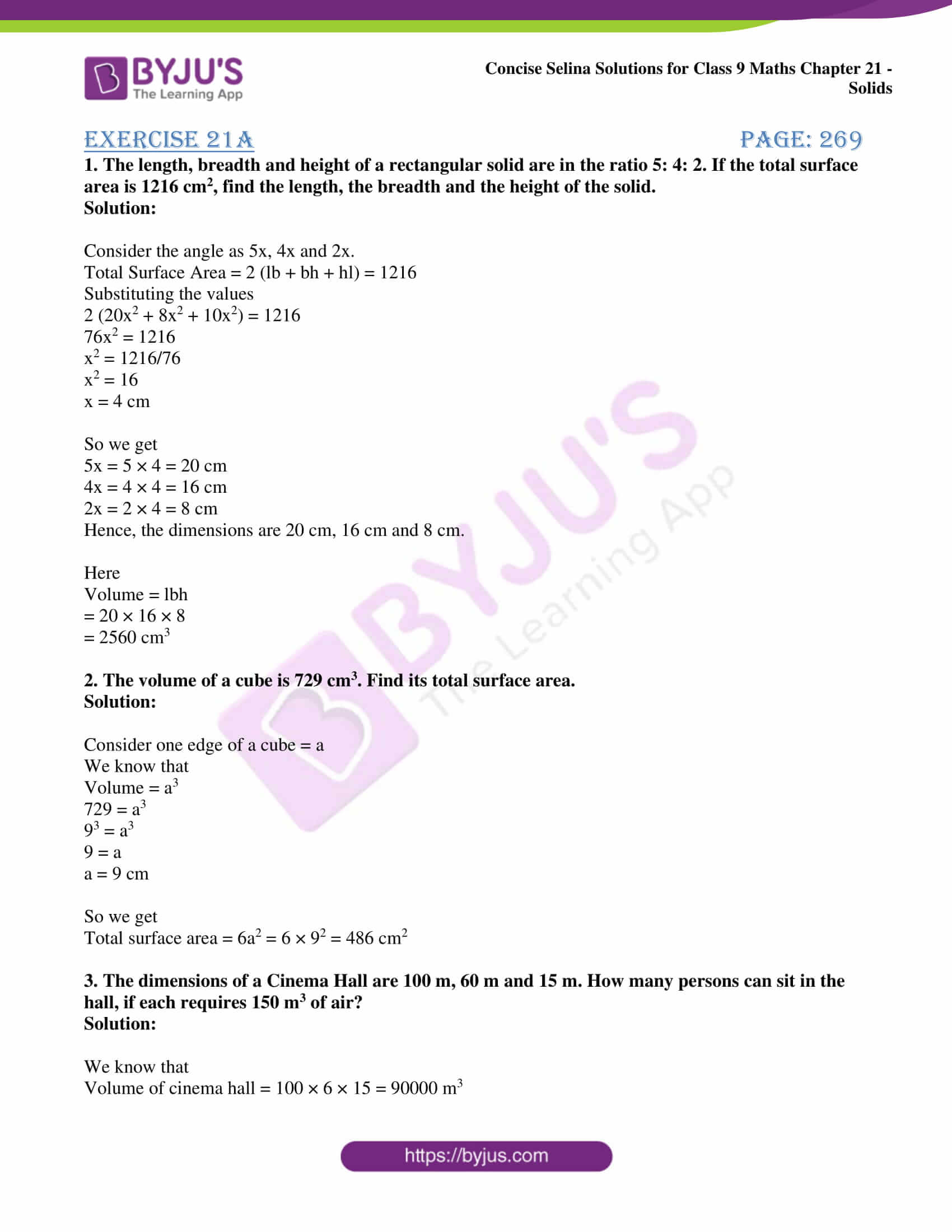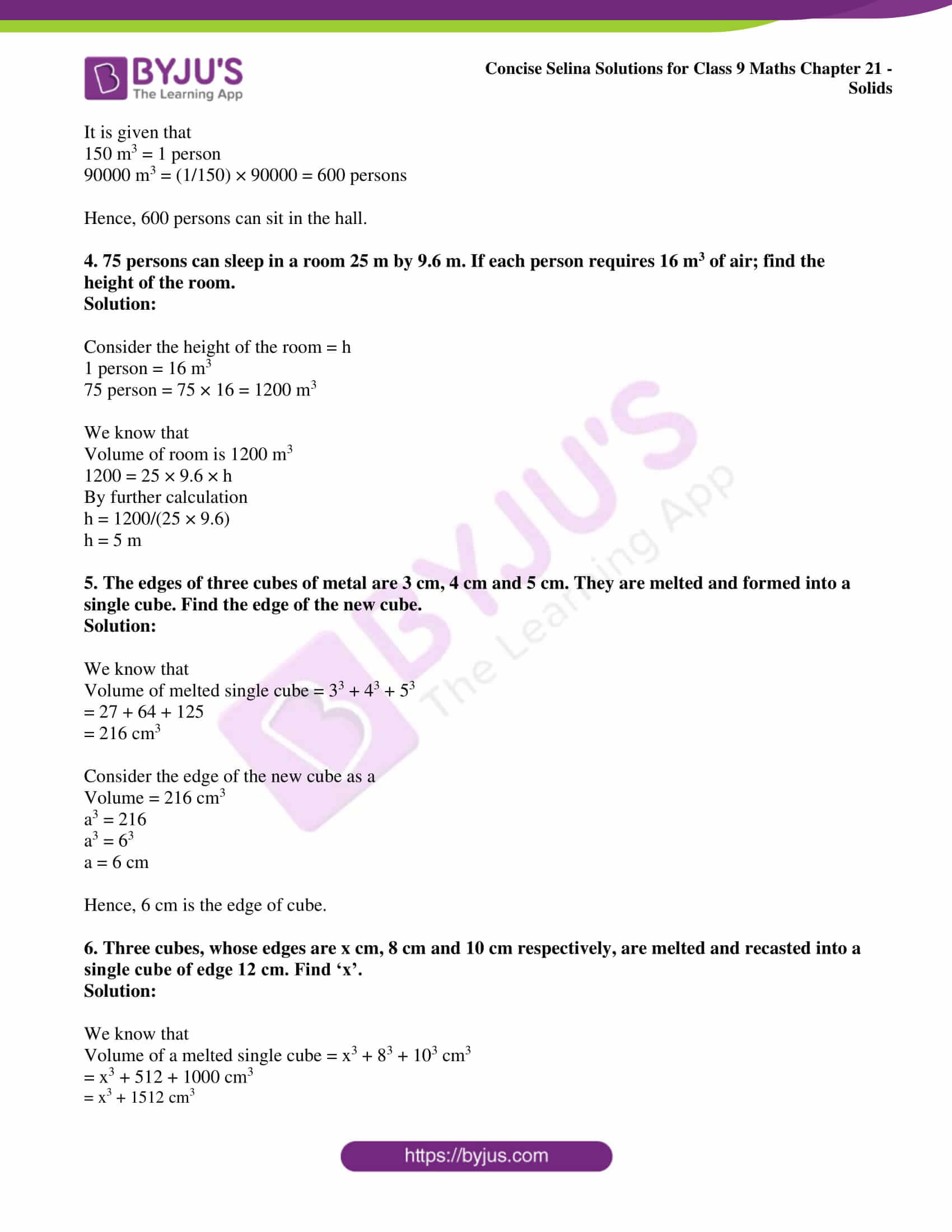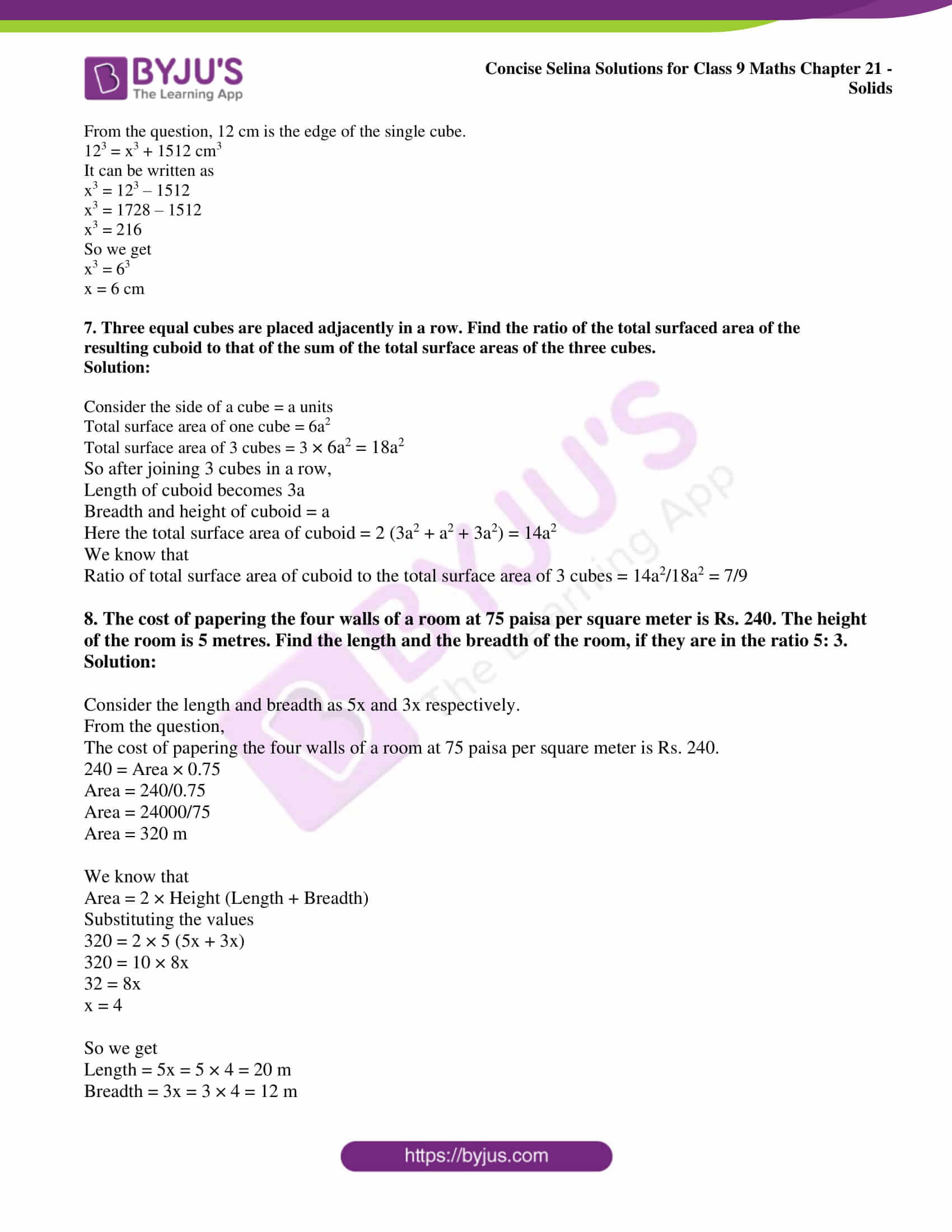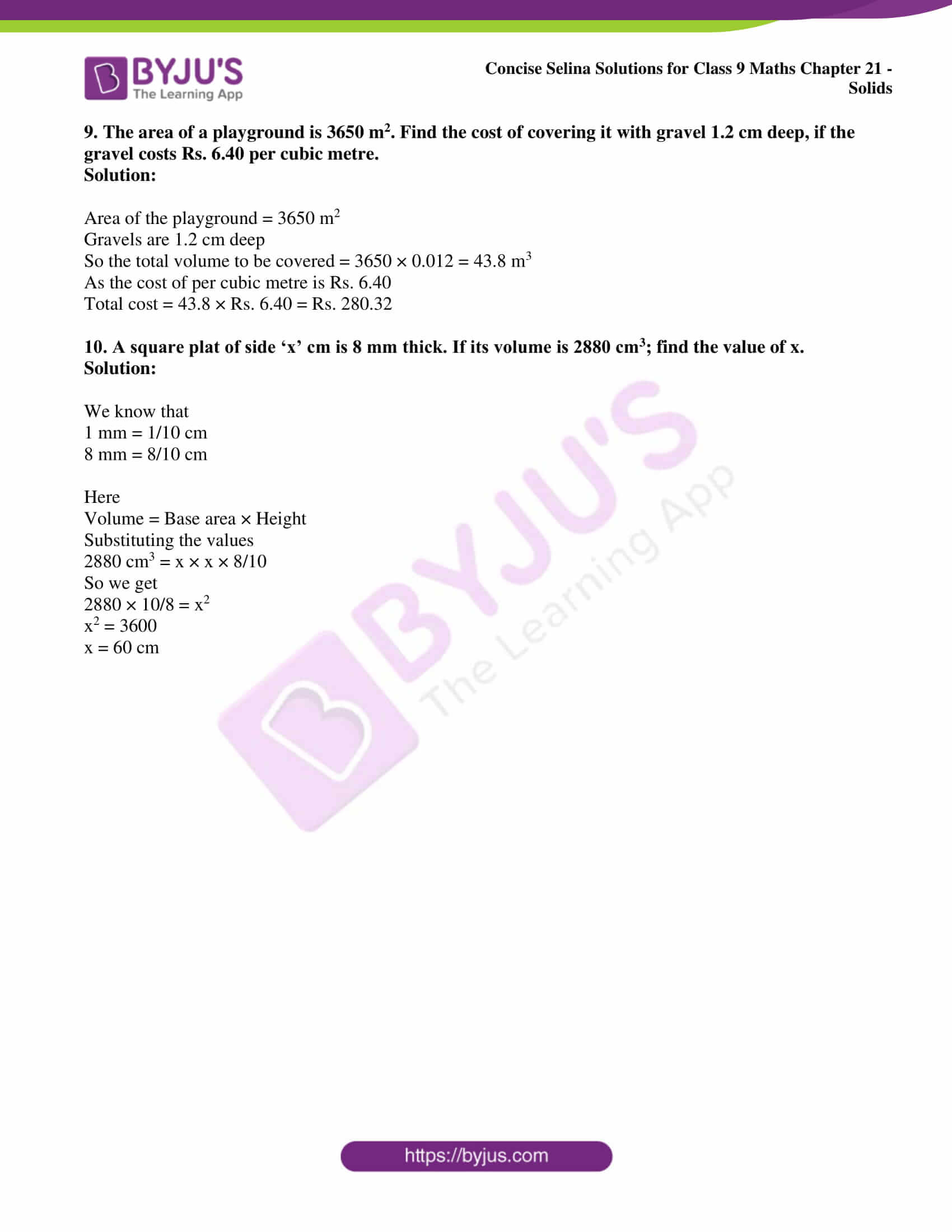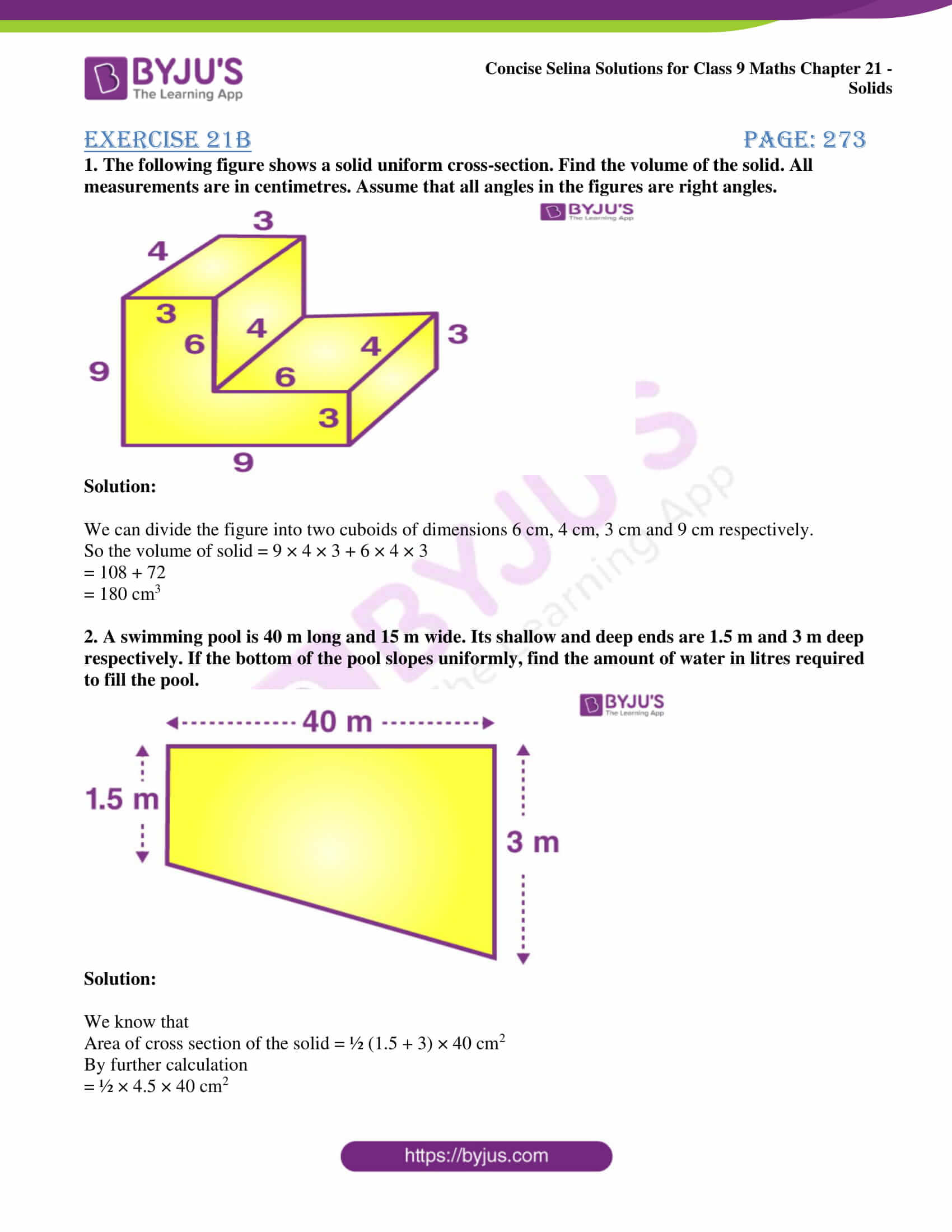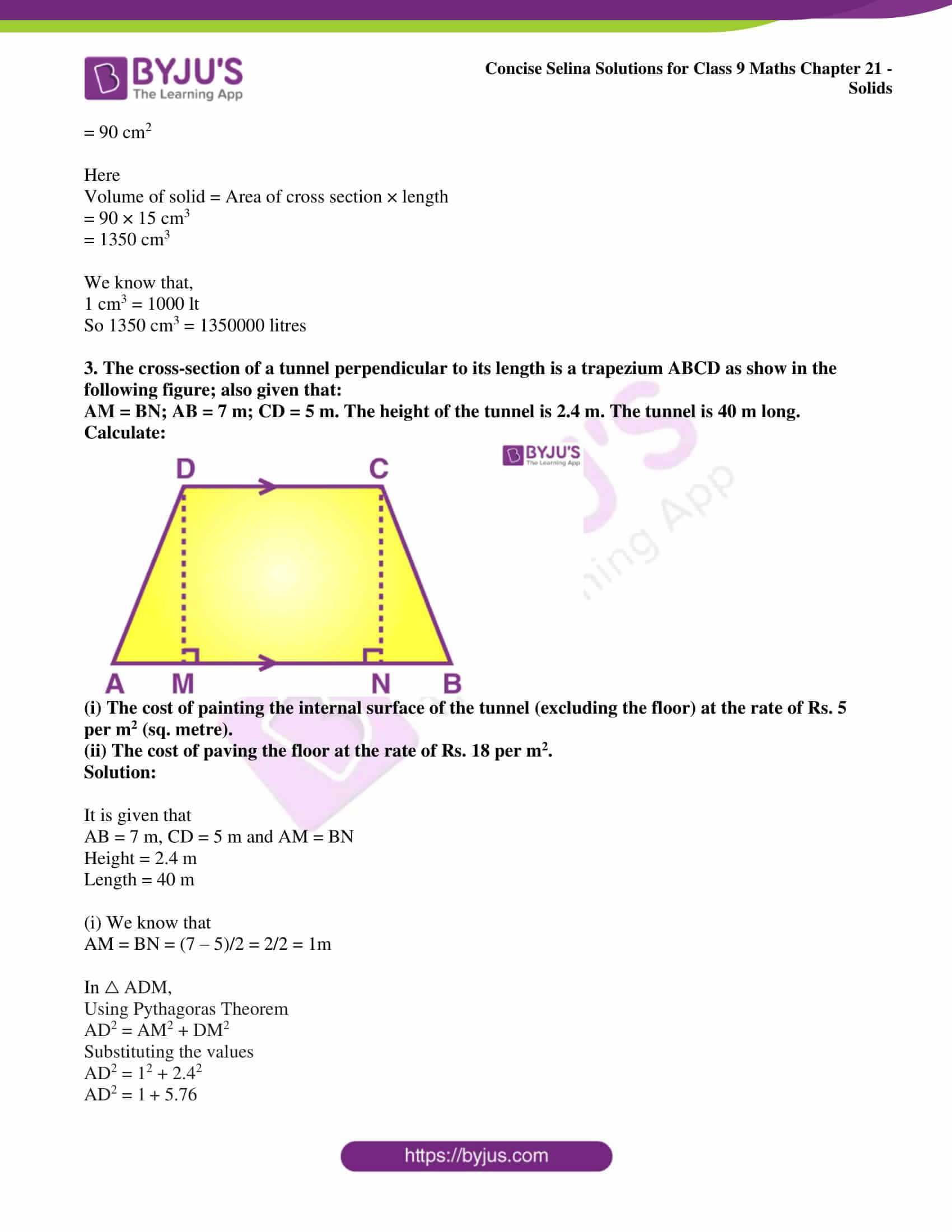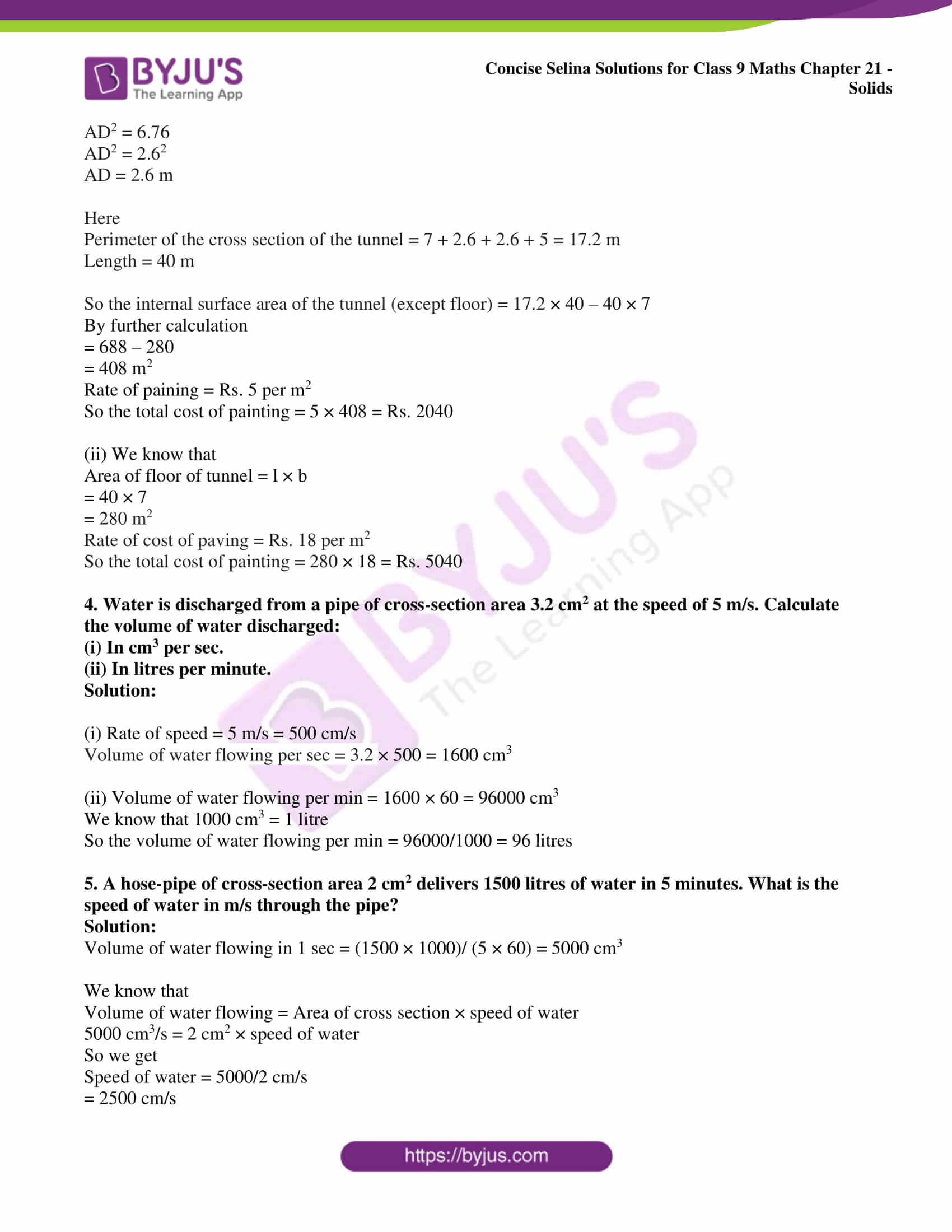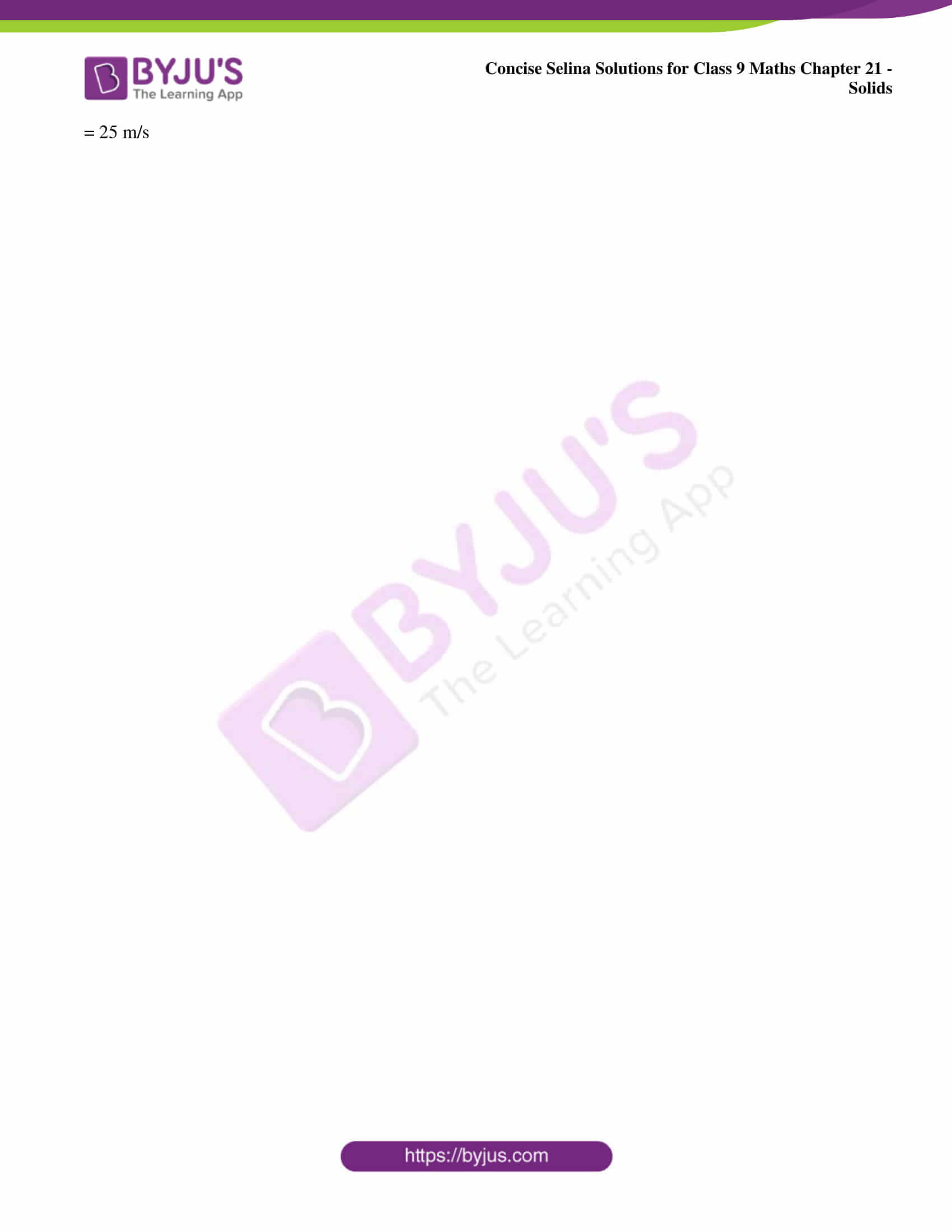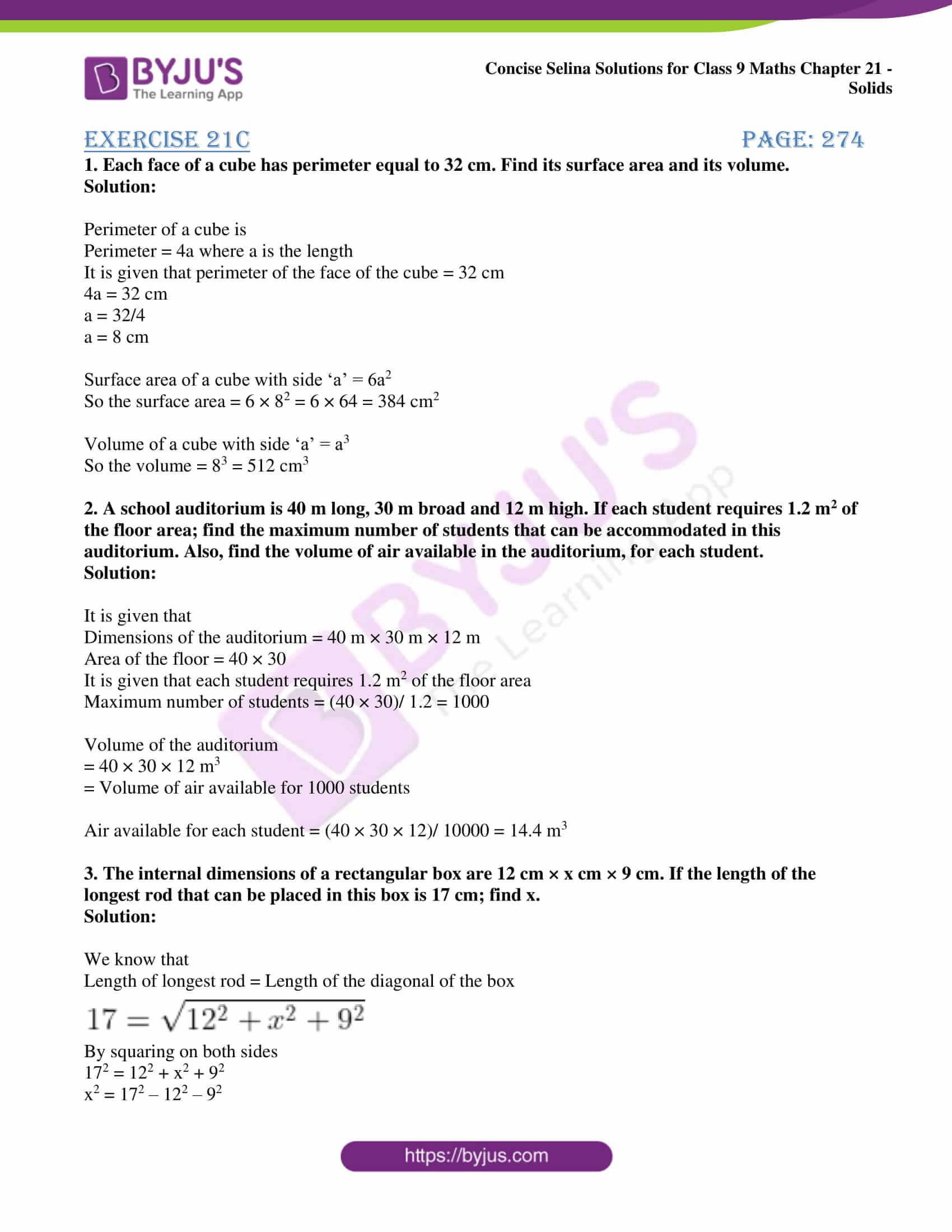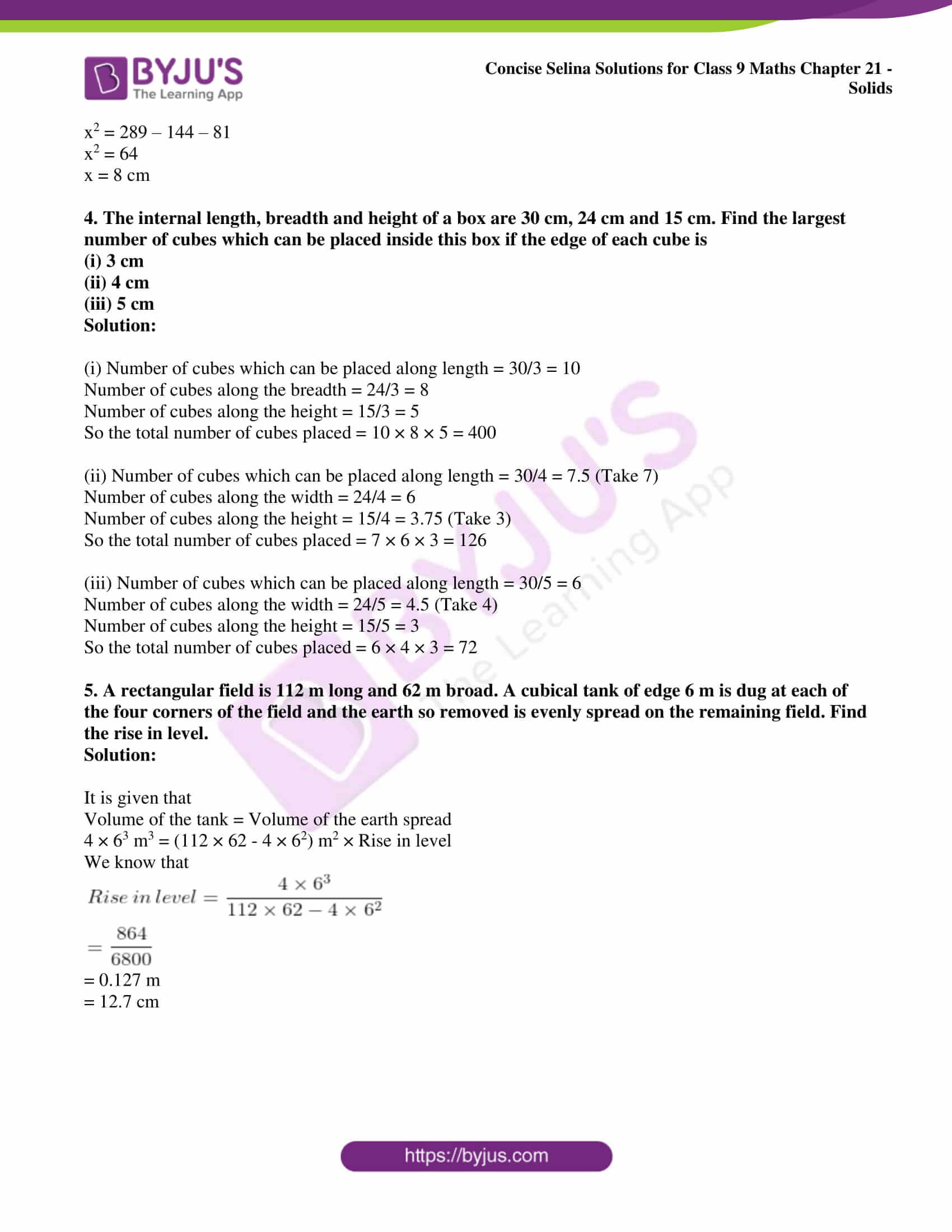Exercise 21A page: 269

1. The length, breadth and height of a rectangular solid are in the ratio 5: 4: 2. If the total surface area is 1216 cm2, find the length, the breadth and the height of the solid.

Solution:

Consider the angle as 5x, 4x and 2x.

Total Surface Area = 2 (lb + bh + hl) = 1216

Substituting the values

2 (20x2 + 8x2 + 10x2) = 1216

76x2 = 1216

x2 = 1216/76

x2 = 16

x = 4 cm

So we get

5x = 5 × 4 = 20 cm

4x = 4 × 4 = 16 cm

2x = 2 × 4 = 8 cm

Hence, the dimensions are 20 cm, 16 cm and 8 cm.

Here

Volume = lbh

= 20 × 16 × 8

= 2560 cm3

2. The volume of a cube is 729 cm3. Find its total surface area.

Solution:

Consider one edge of a cube = a

We know that

Volume = a3

729 = a3

93 = a3

9 = a

a = 9 cm

So we get

Total surface area = 6a2 = 6 × 92 = 486 cm2

3. The dimensions of a Cinema Hall are 100 m, 60 m and 15 m. How many persons can sit in the hall, if each requires 150 m3 of air?

Solution:

We know that

Volume of cinema hall = 100 × 6 × 15 = 90000 m3

It is given that

150 m3 = 1 person

90000 m3 = (1/150) × 90000 = 600 persons

Hence, 600 persons can sit in the hall.

4. 75 persons can sleep in a room 25 m by 9.6 m. If each person requires 16 m3 of air; find the height of the room.

Solution:

Consider the height of the room = h

1 person = 16 m3

75 person = 75 × 16 = 1200 m3

We know that

Volume of room is 1200 m3

1200 = 25 × 9.6 × h

By further calculation

h = 1200/(25 × 9.6)

h = 5 m

5. The edges of three cubes of metal are 3 cm, 4 cm and 5 cm. They are melted and formed into a single cube. Find the edge of the new cube.

Solution:

We know that

Volume of melted single cube = 33 + 43 + 53

= 27 + 64 + 125

= 216 cm3

Consider the edge of the new cube as a

Volume = 216 cm3

a3 = 216

a3 = 63

a = 6 cm

Hence, 6 cm is the edge of cube.

6. Three cubes, whose edges are x cm, 8 cm and 10 cm respectively, are melted and recasted into a single cube of edge 12 cm. Find ‘x’.

Solution:

We know that

Volume of a melted single cube = x3 + 83 + 103 cm3

= x3 + 512 + 1000 cm3

= x3 + 1512 cm3

From the question, 12 cm is the edge of the single cube.

123 = x3 + 1512 cm3

It can be written as

x3 = 123 – 1512

x3 = 1728 – 1512

x3 = 216

So we get

x3 = 63

x = 6 cm

7. Three equal cubes are placed adjacently in a row. Find the ratio of the total surfaced area of the resulting cuboid to that of the sum of the total surface areas of the three cubes.

Solution:

Consider the side of a cube = a units

Total surface area of one cube = 6a2

Total surface area of 3 cubes = 3 × 6a2 = 18a2

So after joining 3 cubes in a row,

Length of cuboid becomes 3a

Breadth and height of cuboid = a

Here the total surface area of cuboid = 2 (3a2 + a2 + 3a2) = 14a2

We know that

Ratio of total surface area of cuboid to the total surface area of 3 cubes = 14a2/18a2 = 7/9

8. The cost of papering the four walls of a room at 75 paisa per square meter is Rs. 240. The height of the room is 5 metres. Find the length and the breadth of the room, if they are in the ratio 5: 3.

Solution:

Consider the length and breadth as 5x and 3x respectively.

From the question,

The cost of papering the four walls of a room at 75 paisa per square meter is Rs. 240.

240 = Area × 0.75

Area = 240/0.75

Area = 24000/75

Area = 320 m

We know that

Area = 2 × Height (Length + Breadth)

Substituting the values

320 = 2 × 5 (5x + 3x)

320 = 10 × 8x

32 = 8x

x = 4

So we get

Length = 5x = 5 × 4 = 20 m

Breadth = 3x = 3 × 4 = 12 m

9. The area of a playground is 3650 m2. Find the cost of covering it with gravel 1.2 cm deep, if the gravel costs Rs. 6.40 per cubic metre.

Solution:

Area of the playground = 3650 m2

Gravels are 1.2 cm deep

So the total volume to be covered = 3650 × 0.012 = 43.8 m3

As the cost of per cubic metre is Rs. 6.40

Total cost = 43.8 × Rs. 6.40 = Rs. 280.32

10. A square plat of side ‘x’ cm is 8 mm thick. If its volume is 2880 cm3; find the value of x.

Solution:

We know that

1 mm = 1/10 cm

8 mm = 8/10 cm

Here

Volume = Base area × Height

Substituting the values

2880 cm3 = x × x × 8/10

So we get

2880 × 10/8 = x2

x2 = 3600

x = 60 cm

Exercise 21B page: 273

1. The following figure shows a solid uniform cross-section. Find the volume of the solid. All measurements are in centimetres. Assume that all angles in the figures are right angles.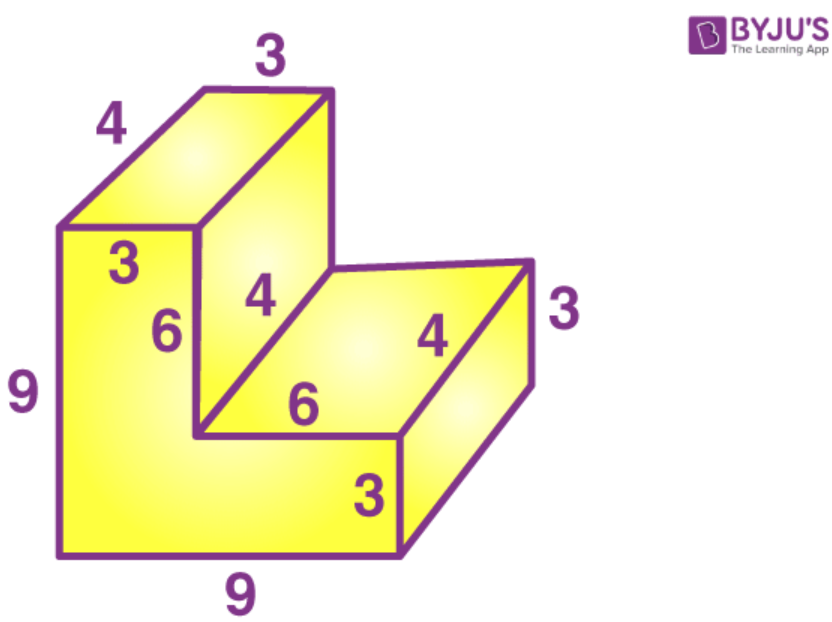Solution:

We can divide the figure into two cuboids of dimensions 6 cm, 4 cm, 3 cm and 9 cm respectively.

So the volume of solid = 9 × 4 × 3 + 6 × 4 × 3

= 108 + 72

= 180 cm3

2. A swimming pool is 40 m long and 15 m wide. Its shallow and deep ends are 1.5 m and 3 m deep respectively. If the bottom of the pool slopes uniformly, find the amount of water in litres required to fill the pool.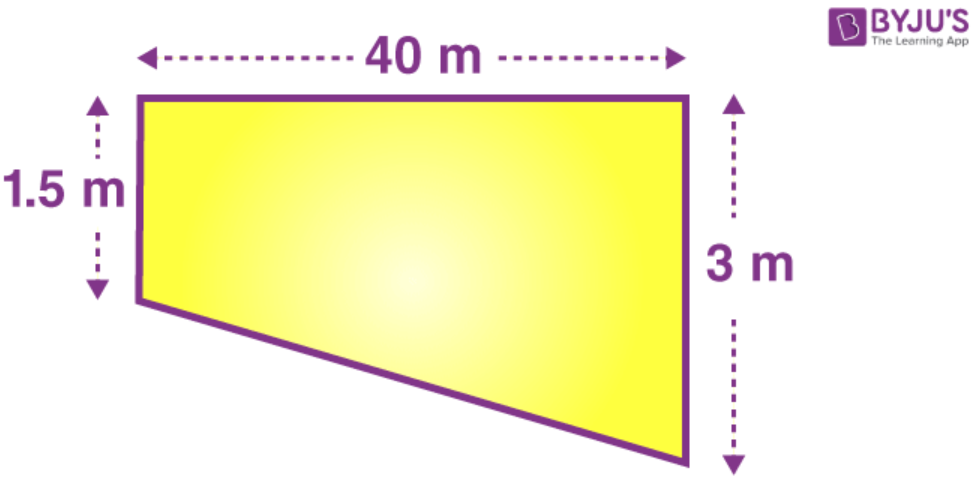Solution:

We know that

Area of cross section of the solid = ½ (1.5 + 3) × 40 cm2

By further calculation

= ½ × 4.5 × 40 cm2

= 90 cm2

Here

Volume of solid = Area of cross section × length

= 90 × 15 cm3

= 1350 cm3

We know that,

1 cm3 = 1000 lt

So 1350 cm3 = 1350000 litres

3. The cross-section of a tunnel perpendicular to its length is a trapezium ABCD as show in the following figure; also given that:

AM = BN; AB = 7 m; CD = 5 m. The height of the tunnel is 2.4 m. The tunnel is 40 m long.

Calculate: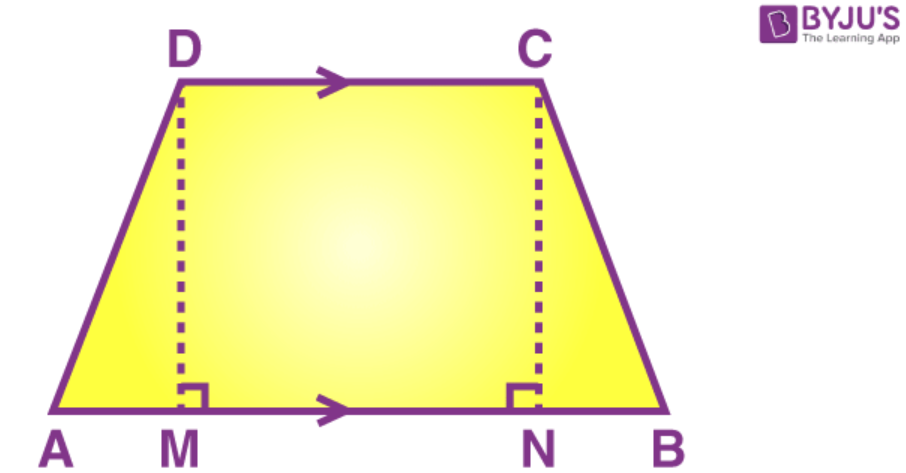(i) The cost of painting the internal surface of the tunnel (excluding the floor) at the rate of Rs. 5 per m2 (sq. metre).

(ii) The cost of paving the floor at the rate of Rs. 18 per m2.

Solution:

It is given that

AB = 7 m, CD = 5 m and AM = BN

Height = 2.4 m

Length = 40 m

(i) We know that

AM = BN = (7 – 5)/2 = 2/2 = 1m

Using Pythagoras Theorem

Substituting the values

Here

Perimeter of the cross section of the tunnel = 7 + 2.6 + 2.6 + 5 = 17.2 m

Length = 40 m

So the internal surface area of the tunnel (except floor) = 17.2 × 40 – 40 × 7

By further calculation

= 688 – 280

= 408 m2

Rate of paining = Rs. 5 per m2

So the total cost of painting = 5 × 408 = Rs. 2040

(ii) We know that

Area of floor of tunnel = l × b

= 40 × 7

= 280 m2

Rate of cost of paving = Rs. 18 per m2

So the total cost of painting = 280 × 18 = Rs. 5040

4. Water is discharged from a pipe of cross-section area 3.2 cm2 at the speed of 5 m/s. Calculate the volume of water discharged:

(i) In cm3 per sec.

(ii) In litres per minute.

Solution:

(i) Rate of speed = 5 m/s = 500 cm/s

Volume of water flowing per sec = 3.2 × 500 = 1600 cm3

(ii) Volume of water flowing per min = 1600 × 60 = 96000 cm3

We know that 1000 cm3 = 1 litre

So the volume of water flowing per min = 96000/1000 = 96 litres

5. A hose-pipe of cross-section area 2 cm2 delivers 1500 litres of water in 5 minutes. What is the speed of water in m/s through the pipe?

Solution:

Volume of water flowing in 1 sec = (1500 × 1000)/ (5 × 60) = 5000 cm3

We know that

Volume of water flowing = Area of cross section × speed of water

5000 cm3/s = 2 cm2 × speed of water

So we get

Speed of water = 5000/2 cm/s

= 2500 cm/s

= 25 m/s

Exercise 21C page: 274

1. Each face of a cube has perimeter equal to 32 cm. Find its surface area and its volume.

Solution:

Perimeter of a cube is

Perimeter = 4a where a is the length

It is given that perimeter of the face of the cube = 32 cm

4a = 32 cm

a = 32/4

a = 8 cm

Surface area of a cube with side ‘a’ = 6a2

So the surface area = 6 × 82 = 6 × 64 = 384 cm2

Volume of a cube with side ‘a’ = a3

So the volume = 83 = 512 cm3

2. A school auditorium is 40 m long, 30 m broad and 12 m high. If each student requires 1.2 m2 of the floor area; find the maximum number of students that can be accommodated in this auditorium. Also, find the volume of air available in the auditorium, for each student.

Solution:

It is given that

Dimensions of the auditorium = 40 m × 30 m × 12 m

Area of the floor = 40 × 30

It is given that each student requires 1.2 m2 of the floor area

Maximum number of students = (40 × 30)/ 1.2 = 1000

Volume of the auditorium

= 40 × 30 × 12 m3

= Volume of air available for 1000 students

Air available for each student = (40 × 30 × 12)/ 10000 = 14.4 m3

3. The internal dimensions of a rectangular box are 12 cm × x cm × 9 cm. If the length of the longest rod that can be placed in this box is 17 cm; find x.

Solution:

We know that

Length of longest rod = Length of the diagonal of the box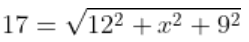By squaring on both sides

172 = 122 + x2 + 92

x2 = 172 – 122 – 92

x2 = 289 – 144 – 81

x2 = 64

x = 8 cm

4. The internal length, breadth and height of a box are 30 cm, 24 cm and 15 cm. Find the largest number of cubes which can be placed inside this box if the edge of each cube is

(i) 3 cm

(ii) 4 cm

(iii) 5 cm

Solution:

(i) Number of cubes which can be placed along length = 30/3 = 10

Number of cubes along the breadth = 24/3 = 8

Number of cubes along the height = 15/3 = 5

So the total number of cubes placed = 10 × 8 × 5 = 400

(ii) Number of cubes which can be placed along length = 30/4 = 7.5 (Take 7)

Number of cubes along the width = 24/4 = 6

Number of cubes along the height = 15/4 = 3.75 (Take 3)

So the total number of cubes placed = 7 × 6 × 3 = 126

(iii) Number of cubes which can be placed along length = 30/5 = 6

Number of cubes along the width = 24/5 = 4.5 (Take 4)

Number of cubes along the height = 15/5 = 3

So the total number of cubes placed = 6 × 4 × 3 = 72

5. A rectangular field is 112 m long and 62 m broad. A cubical tank of edge 6 m is dug at each of the four corners of the field and the earth so removed is evenly spread on the remaining field. Find the rise in level.

Solution:

It is given that

Volume of the tank = Volume of the earth spread

4 × 63 m3 = (112 × 62 – 4 × 62) m2 × Rise in level

We know that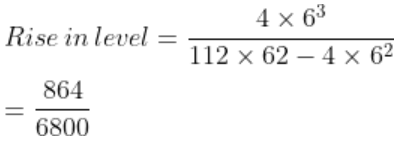= 0.127 m

= 12.7 cm

## Selina Solutions for Class 9 Maths Chapter 21- Solids

Chapter 21, Solids, is composed of 3 exercises and the solutions given here contain answers to all the questions present in this exercise. Let us have a look at some of the topics that are being discussed in this chapter.

21.1 Introduction

21.2 Cuboid

21.3 Cube

21.4 Cost of an article

21.5 Cross-section

21.6 Flow of water

## Selina Solutions for Class 9 Maths Chapter 21- Solids

Anything which occupies space and has a definite shape is called a solid. The space occupied by a solid is called its volume. The sum of the areas of all the surfaces of a solid is called its surface area or its total surface area. Read and learn Chapter 21 of Selina textbook to familiarize with the concepts related to Solids. Learn the Selina Solutions for Class 9 effectively to score high in the examination.